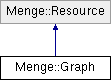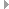Menge Modular Pedestrian Simulation Framework for Research and Development
Menge::Graph Class Reference

A roadmap graph and the infrastructure for performing graph searches. NOTE: This implementation assumes that the graph doesn't change. More...

`#include <Graph.h>`

Inheritance diagram for Menge::Graph:## Public Member Functions

Graph (const std::string &fileName)
Constructor. More...

virtual const std::string & getLabel () const
Returns a unique resource label to be used to identify different resource types which use the same underlying file data.

void clear ()
Clears the graph – such that it has no vertices and no edges.

RoadMapPathgetPath (const Agents::BaseAgent *agent, const BFSM::Goal *goal)
Compute path. More...

size_t getVertexCount () const
Return the number of vertices in the graph. More...

const GraphVertexgetVertex (size_t i) const
Returns a const pointer to the ith vertex. More...Public Member Functions inherited from Menge::Resource
Resource (const std::string &fileName)
Constructor. More...

void destroy ()
This supplants the destructor. More...

const std::string & getName () const
Return the file name for this resource. More...

int incRef ()
Increment references to the managed data. More...

int decRef ()
Decrement references to the managed data. More...

bool isUnreferenced () const
Reports if the data is referenced. More...

## Static Public Member Functions

Parses a graph definition and returns a pointer to it. More...

## Static Public Attributes

static const std::string LABEL
The unique label for this data type to be used with resource management.

## Protected Member Functions

~Graph ()
Destructor.

size_t getClosestVertex (const Vector2 &point, float radius)
Find the closest visible graph vertex to the given point. More...

Computes the shortest path from start to end vertices. More...

float computeH (size_t v, const Vector2 &goal)
Compute's "h" for the A* algorithm. H is the estimate of the cost of a node to a goal point. In this case, simply Euclidian distance. More...

void initHeapMemory ()
Initializes the heap memory based on current graph state.Protected Member Functions inherited from Menge::Resource
virtual ~Resource ()
Virtual destructor.

## Protected Attributes

size_t _vCount
The number of vertices.

GraphVertex_vertices
An array containing all vertices.

size_t DATA_SIZE
The size of a block of data used for COST in the A* algorithm (3N, N = number of nodes)

size_t STATE_SIZE
The size of a block of data used for STATE in the A* algorithm (2N, N = number of nodes)

unsigned int * _HEAP
The full set of data to serve as the heap There are N entries in a single heap and one heap per thread.

unsigned int * _PATH
The full set of data for reconstructing the path. For any given entry i, the value at i is the index of the node from which i was reached. There is one block per thread.

float * _DATA
The block of data for tracking the f, g, and h data for nodes. (This is where DATA_SIZE = 3N comes from.) One block for each active thread. The first N values in a block are the f values, the next N are the g values, and the last set of N values are the h values.

bool * _STATE
Block of data for reportin node state (if its in a heap or if its no longer used for calculation. First N booleans are "in heap", second N are "finished". One block of 2N booleans per thread.Protected Attributes inherited from Menge::Resource
const std::string _fileName
The file which contains the resource's data.

int _refCount
The number of data wrappers using this managed data.

SimpleLock _lock
Simple lock to handle reference counts safely.

## Detailed Description

A roadmap graph and the infrastructure for performing graph searches. NOTE: This implementation assumes that the graph doesn't change.

## Constructor & Destructor Documentation

 Menge::Graph::Graph ( const std::string & fileName )

Constructor.

Parameters
 fileName The name of the file which contains the vector field definition.

## Member Function Documentation

 float Menge::Graph::computeH ( size_t v, const Vector2 & goal )
inlineprotected

Compute's "h" for the A* algorithm. H is the estimate of the cost of a node to a goal point. In this case, simply Euclidian distance.

Parameters
 v The vertex to estimate the cost. goal The goal point.
Returns
The h-value.
 size_t Menge::Graph::getClosestVertex ( const Vector2 & point, float radius )
protected

Find the closest visible graph vertex to the given point.

Parameters
 point The point to connect to the graph. radius The radius of the agent testing.
Returns
The index of the closest node.
 RoadMapPath * Menge::Graph::getPath ( const Agents::BaseAgent * agent, const BFSM::Goal * goal )

Compute path.

Parameters
 agent The agent for whom to compute the path. goal The agent's goal.
Returns
A pointer to a RoadMapPath. If there is an error, the poitner will be NULL.
 RoadMapPath * Menge::Graph::getPath ( size_t startID, size_t endID )
protected

Computes the shortest path from start to end vertices.

This function instantiates a new path, but the caller is responsible for deleting it.

Parameters
 startID The index of the start vertex. endID The index of the end vertex.
Returns
A pointer to a new RoadMapPath.
 const GraphVertex * Menge::Graph::getVertex ( size_t i ) const

Returns a const pointer to the ith vertex.

The validitiy of the index is only checked in debug mode with an assertion.

Parameters
 i The index of the desired pointer.
Returns
A const pointer to the ith graph vertex.
 size_t Menge::Graph::getVertexCount ( ) const
inline

Return the number of vertices in the graph.

Returns
The number of vertices in the graph.
 Resource * Menge::Graph::load ( const std::string & fileName )
static

Parses a graph definition and returns a pointer to it.

This function works in conjunction with the ResourceManager. That is why it returns a pointer, not to a Graph, but to a Resource. The ResourceManager uses it to load and instantiate Graph instances.

Parameters
 fileName The path to the file containing the VectorField definition.
Returns
A pointer to the new Graph (if the file is valid), NULL if invalid.

The documentation for this class was generated from the following files:
• src/menge/MengeCore/resources/Graph.h
• src/menge/MengeCore/resources/Graph.cpp